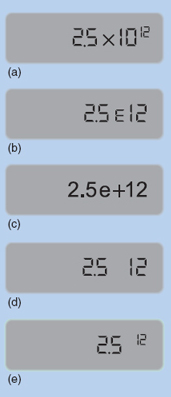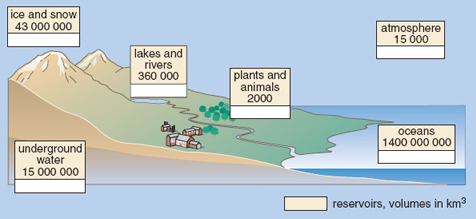Water for life

Start this free course now. Just create an account and sign in. Enrol and complete the course for a free statement of participation or digital badge if available.

Free course

# 2.2.1 Using a calculator for scientific notation

You are likely to be doing many calculations with numbers in scientific notation, so it is important that you know how to input them on your calculator efficiently and how to interpret the results.

First, make sure that you can input numbers in scientific notation on your calculator. There are a couple of ways to do this, but the most straightforward is to use the special button provided for entering scientific notation. This might be labelled as EXP, EE, E or EX, but there is considerable variation between calculators. Make sure that you can find the appropriate button on your calculator. Using this sort of button is equivalent to typing the whole of '× 10 to the power'. So, on a particular calculator, keying 2.5 EXP 12 enters all of 2.5 × 1012.

To enter a number such as 109 on your calculator using the scientific notation button, it is helpful to remember that 109 is written as 1 × 109 in scientific notation, so you will need to key in for example 1 EXP 9.

In addition to being able to enter numbers in scientific notation on your calculator, it is important that you can understand your calculator display when it gives an answer in scientific notation. Enter the number 2.5 × 1012 on your calculator and look at the display. Again there is considerable variation among calculators, but the display will probably be similar to one of those shown in Figure 3. The 12 at the right of the display is the power of ten, but note that the ten itself is frequently not displayed. If your calculator displays 2.5 × 1012 as shown in Figure 3(e), you will need to take particular care; this does not mean 2.512 on this occasion. You should be careful not to copy down a number displayed in this way on your calculator as an answer to a question; this could cause confusion at a later stage. No matter how scientific notation is entered and displayed on your calculator or computer, when writing it on paper you should always use the form exemplified by 2.5 × 1012.Figure 3 Examples of how various calculators display the number 2.5 × 1012

## Question 4

To check that you can use your calculator for scientific notation, do the following calculations.

(a) (4.5 × 104) × (4.0 × 1011)

(b) 1012 − (5.66 × 1011)

(a) (4.5 × 104) × (4.0 × 1011) = 1.8 × 1016

(b) 1012 − (5.66 × 1011) = 4.34 × 1011

(Note: if you got the incorrect answer 9.434 × 1012 you probably entered 10 EXP 12 instead of 1 EXP 12 on your calculator. Remember that 1012 can be written as 1 × 1012.)

## Question 5

The numbers in Table 1 can be presented in a different way. In Figure 4 (below) they are given more visual interest by being displayed in a diagram that shows the locations of the reservoirs as well. The amounts of water stored in the various natural reservoirs are shown in the boxes.Figure 4 The amounts of water (in km3) stored within the Earth's natural reservoirs

Convert each number in Figure 4 into correct scientific notation, and note down your answers.

Your answers should be as follows: ice and snow, 4.3 × 107; underground water, 1.5 × 107; lakes and rivers, 3.6 × 105; plants and animals, 2.0 × 103; atmosphere, 1.5 × 104; oceans, 1.4 × 109.

S154_1